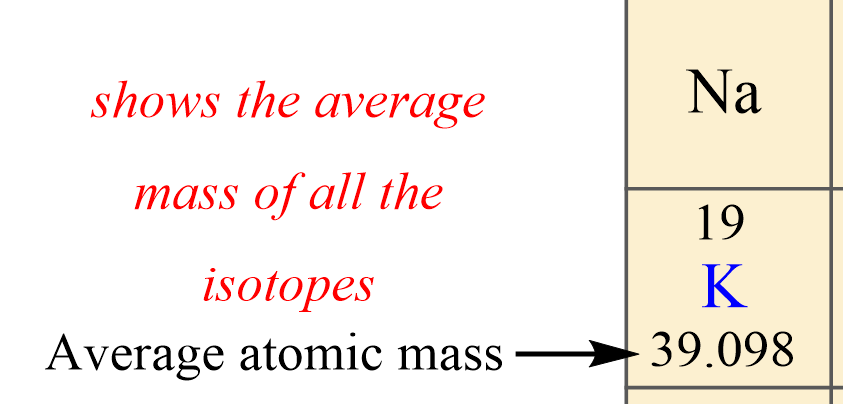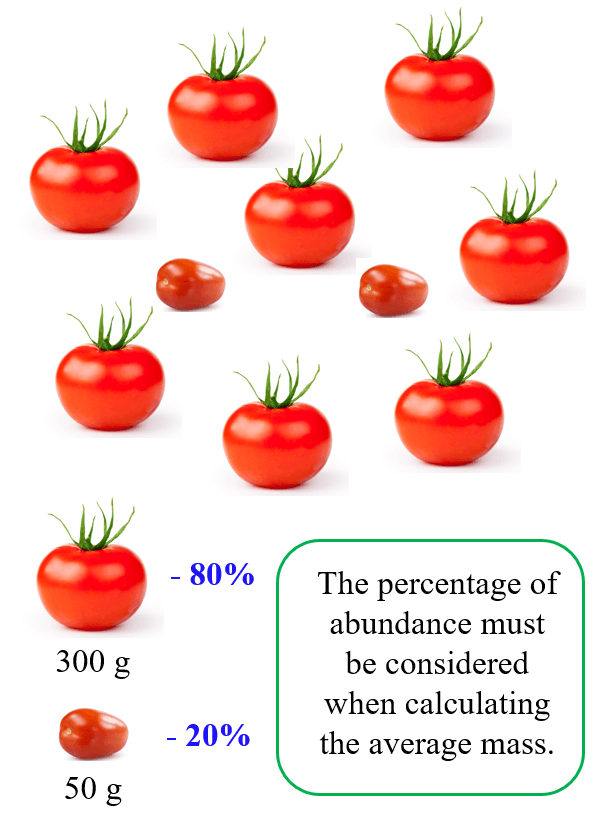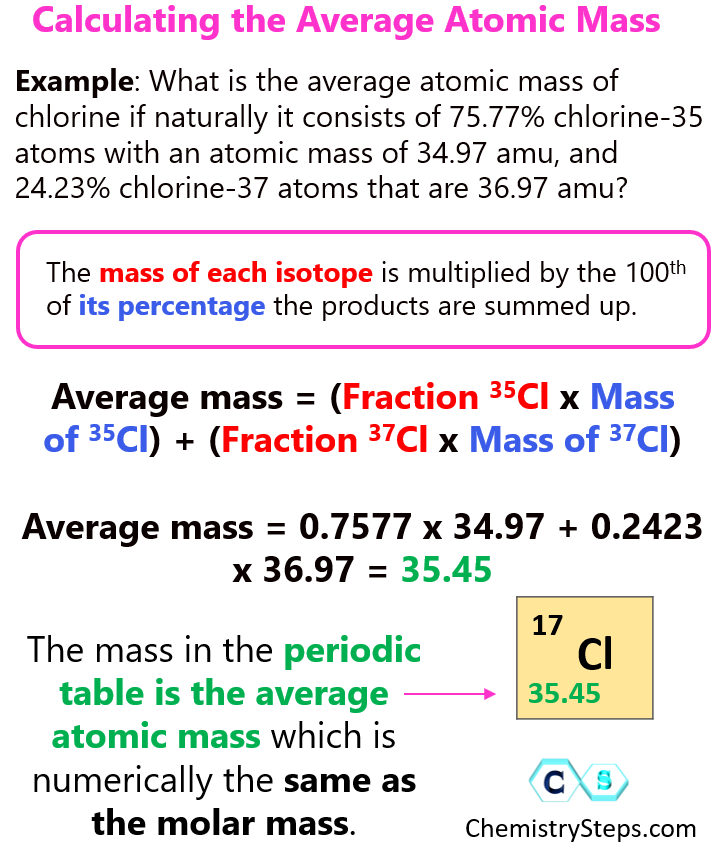## Atoms, Molecules, and Ions

In the previous post, when talking about isotopes, we mentioned that the mass given for elements in the preperiodic table is not a whole number. For example, the mass of potassium is given as 39.098, and this is because that number is the average atomic mass:The average atomic mass is the average mass of all the isotopes that compose that element, weighted based on the natural abundance of each isotope.

# So, how do we calculate the average atomic mass?

Let’s see this by doing an example on chlorine. Naturally occurring chlorine consists of 75.77% chlorine-35 atoms with an atomic mass of 34.97 amu, and 24.23% chlorine-37 atoms that are 36.97 amu.

It may seem that we can simply add the two numbers and divide the product by 2 and get the average atomic mass. However, this would be correct if we had equal amounts of each isotope.

For example, let’s say we have two types of tomatoes: large and small:Now, if 8 out of 10 of these tomatoes are large, then we cannot say that the average mass of the tomatoes is (300 + 50)/2 = 175 g.

This would be the average mass of just two tomatoes, or of any sample of equal quantity. However, if we want to know the average mass of all the tomatoes, we need to know how many percent of each we have.For this example, we have 80% large and 20% small tomatoes, therefore, to calculate the average mass, we multiply the mass of each tomato by the 100th fraction of its percentage:

Average mass = (% large) x (mass large) + (% small) x (mass small)

Average mass = 0.8 x 300 + 0.2 x 50 = 250 g

So, the average mass is closer to the mass of the larger tomato because it is more abundant.

Now, we do the same thing for atoms. The mass of each isotope is multiplied by its percentage the products are summed up. Therefore, for chlorine, we’d have:

Average mass = (Fraction 35Cl x Mass of 35Cl) + (Fraction 37Cl x Mass of 37Cl)

Average mass = 0.7577 x 34.97 + 0.2423 x 36.97 = 35.45

Notice that the average atomic mass is closer to 35 because the isotope with an atomic mass of 35 is more abundant in nature.

Let’s do one more example.

What is the average atomic mass of carbon if it consists of two major isotopes: 98.89% of 12C and 1.11% of 13C.

Average mass = (Fraction 12C x Mass of 12C) + (Fraction 13C x Mass of 13C)

Average mass = 0.9889 x 12 + 0.0111 x 13.0034 = 12.011

And this is the number for the average atomic mass of carbon that will be given in the periodic.

To summarize, were is how the average atomic mass is calculated:More practice examples on average atomic mass and isotopes can be found in this multiple-choice quiz:

Check Also